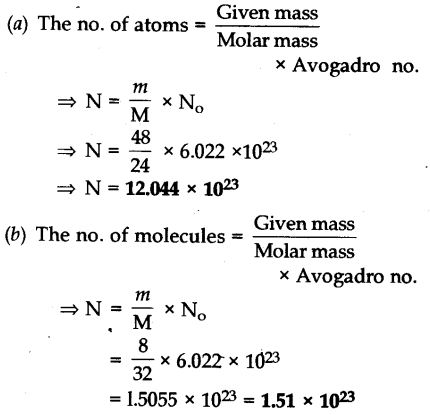# Calculate the number of particles in each of the following: (a) 48 g of Mg (b) 8 g of O2 (c) 0.1 mole of carbon (Atomic mass Mg = 24 u, O = 16 u, C = 12 u, Avogadro constant (NA = 6.022 x 10^23)© The no. of particles (atom) = no. of moles of particles x Avogadro no.
= 0.1 x 6.022 x 10^23
= 6.022 x 10^22